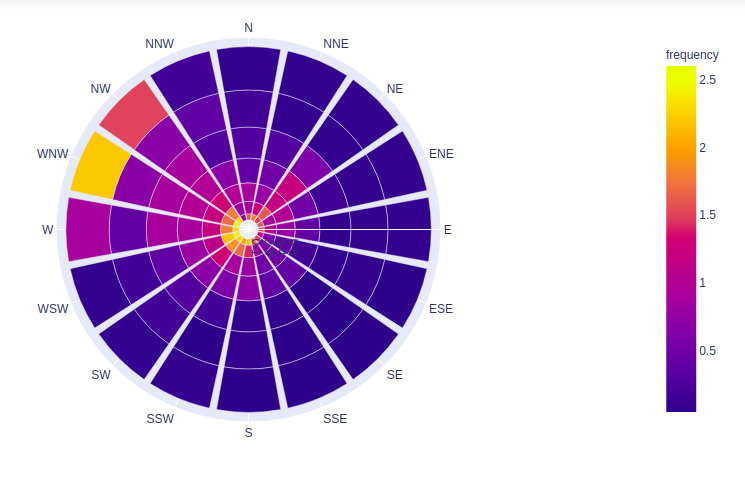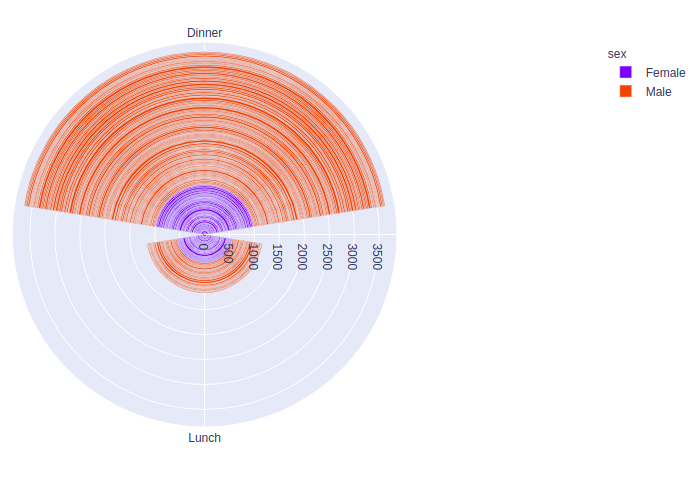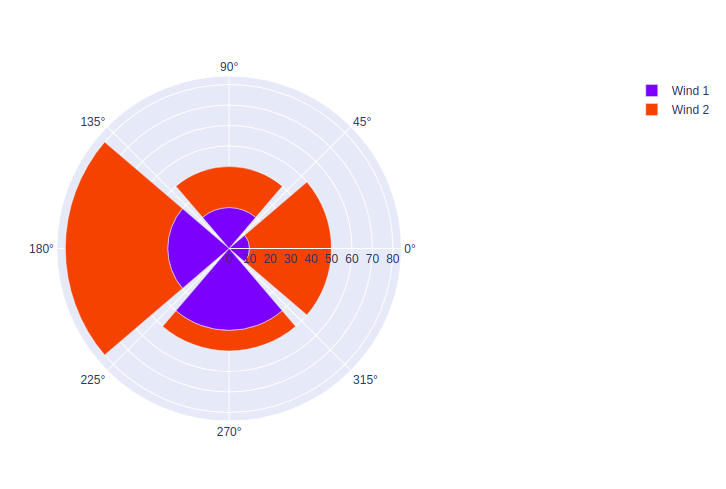Related Articles

# How to make Wind Rose and Polar Bar Charts in Plotly – Python?

• Last Updated : 01 Oct, 2020

A Plotly is a Python library that is used to design graphs, especially interactive graphs. It can plot various graphs and charts like histogram, barplot, boxplot, spreadplot, and many more. It is mainly used in data analysis as well as financial analysis. plotly is an interactive visualization library.

## What is Wind Rose and Polar Bar Charts?

The wind rose chart are graphical charts that show the speed and direction of winds at a location over a period of time. This chart is represented in circular format and the circle indicates the amount of time that the wind blows from a particular direction. The wind rose chart is also known as the polar bar chart.

### Creating Wind Rose Chart

In plotly, a wind rose chart can be made by using the go.Barpolar() method of graph_object class or by px.bar_polar() method of express class.

Syntax: plotly.graph_objects.Barpolar(arg=None,dr=None, dtheta=None,r=None,theta=None)

Syntax: plotly.express.bar_polar(data_frame=None, r=None, theta=None, color=None,)

Example 1:

## Python3

 `import` `plotly.express as px ` ` `  `# using the wind dataset ` `df ``=` `px.data.wind() ` ` `  `fig ``=` `px.bar_polar(df, r``=``"strength"``, theta``=``"direction"``, ` `                   ``color``=``"frequency"``,) ` `fig.show()`

Output:Example 2:

## Python3

 `import` `plotly.express as px ` ` `  ` `  `df ``=` `px.data.tips() ` ` `  `fig ``=` `px.bar_polar(df, r``=``"total_bill"``, theta``=``"time"``, ` `                   ``color``=``"sex"``,) ` ` `  `fig.show()`

Output:Example 3:

## Python3

 `import` `plotly.graph_objects as go ` ` `  `fig ``=` `go.Figure() ` ` `  `fig.add_trace(go.Barpolar( ` `    ``r``=``[``10``, ``20``, ``30``, ``40``], ` `    ``name``=``'Wind 1'``, ` `)) ` ` `  `fig.add_trace(go.Barpolar( ` `    ``r``=``[``40``, ``20``, ``50``, ``10``], ` `    ``name``=``'Wind 2'``, ` `)) ` ` `  `fig.show()`

Output:Attention geek! Strengthen your foundations with the Python Programming Foundation Course and learn the basics.

To begin with, your interview preparations Enhance your Data Structures concepts with the Python DS Course. And to begin with your Machine Learning Journey, join the Machine Learning – Basic Level Course

My Personal Notes arrow_drop_up
Recommended Articles
Page :